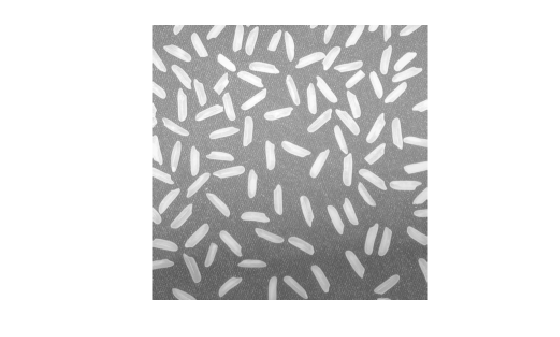## Syntax

``Z = imadd(X,Y)``

## Description

example

````Z = imadd(X,Y)` adds each element in array `X` with the corresponding element in array `Y` and returns the sum in the corresponding element of the output array `Z`.```

## Examples

collapse all

This example shows how to add two `uint8` arrays with truncation for values that exceed 255.

```X = uint8([ 255 0 75; 44 225 100]); Y = uint8([ 50 50 50; 50 50 50 ]); Z = imadd(X,Y)```
```Z = 2x3 uint8 matrix 255 50 125 94 255 150 ```

Read two grayscale `uint8` images into the workspace.

```I = imread('rice.png'); J = imread('cameraman.tif');```

Add the images. Specify the output as type `uint16` to avoid truncating the result.

`K = imadd(I,J,'uint16');`

Display the result.

`imshow(K,[])`Read an image into the workspace.

`I = imread('rice.png');`

Add a constant to the image.

`J = imadd(I,50);`

Display the original image and the result.

`imshow(I)````figure imshow(J)```## Input Arguments

collapse all

First array, specified as a numeric array or logical array of any dimension.

Second array to be added to `X`, specified as a numeric or logical array of the same size and class as `X`, or a numeric scalar of type `double`.

## Output Arguments

collapse all

Sum, returned as a numeric array of the same size as `X`. `Z` is the same class as `X` unless `X` is logical, in which case `Z` is data type `double`. If `X` is an integer array, elements of the output that exceed the range of the integer type are truncated, and fractional values are rounded.

## Version History

Introduced before R2006a